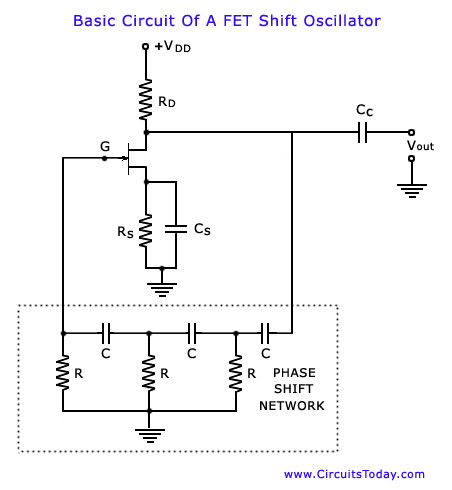## Audio

[Audio][slideshow]We select the so called phase shift oscillator as a first example as it exemplifies very simply the principles set forth in the previous blog post. The circuit is drawn to show clearly the amplifier and feedback network. The circuit consists of a common source FET amplifier followed by a three section R-C phase shift network. The amplifier stage is self-biased with a capacitor bypassed source resistor Rs and a drain bias resistance RD. The output of the last section is supplied back to the gate. If the loading of the phase-shift network on the amplifier can be assumed to be negligible, a phase shift of 180° between the amplified output voltage Vout and the input voltage Vin at the gate is produced by the amplifier itself. The three-section R-C phase shift network produces an additional phase shift, which is a function of frequency and equals 180° at some frequency of operation. At this frequency the total phase shift from the gate around the circuit and back to gate will be exactly zero. This particular frequency will be the one at which the circuit will oscillate provided that the magnitude of the amplification is sufficiently large. In a FET phase-shift oscillator voltage series feedback [that is, feedback voltage proportional to the output voltage Vout and supplied in series with the input signal at the gate is used.

The frequency of the oscillator output depends upon the values of capacitors C and re­sistors R used in the phase shift network. Using basic RC circuit analysis technique, it can be shown that the network phase shift is 180° when

Xc = √6 R or 1 / 2∏fc = √6 R or f = 1 / / 2∏ R c √6

The frequency can be adjusted over a wide range if variable capacitors are used.As well as phase shifting, the R-C network attenuates the amplifier output. Network analysis shows that when the necessary phase shift of 180° is obtained, this network attenuates the output voltage by a factor of 1/29. This means that the amplifier must have a voltage gain of 29 or more. When the amplifier voltage gain is 29 and feedback factor of R-C network, β= 1/29 then the loop gain is β A = 1, the amplifier phase shift of – 180° combined with the network phase shift of + 180° gives a loop phase shift of zero. Both of these conditions are necessary to satisfy the Barkhausen criteria. If the amplifier gain is much greater than 29, the oscillator output waveform is likely to be distorted. When the gain is slightly greater than 29, the output is usually a reasonably pure sinusoidal.

The advantages and disadvantages of phase shift oscillators are given below :

• It is cheap and simple circuit as it contains resistors and capacitors (not bulky and expensive high-value inductors).
• It provides good frequency stability.
• The phase shift oscillator circuit is much simpler than the Wien bridge oscillator circuit because it does not need negative feedback and the stabilization arrangements.
• The output is sinusoidal that is quite distortion free.
• They have a wide frequency range (from a few Hz to several hundred kHz).
• They are particularly suitable for low frequencies, say of the order of 1 Hz, as these frequencies can be easily obtained by using R and C of large values.